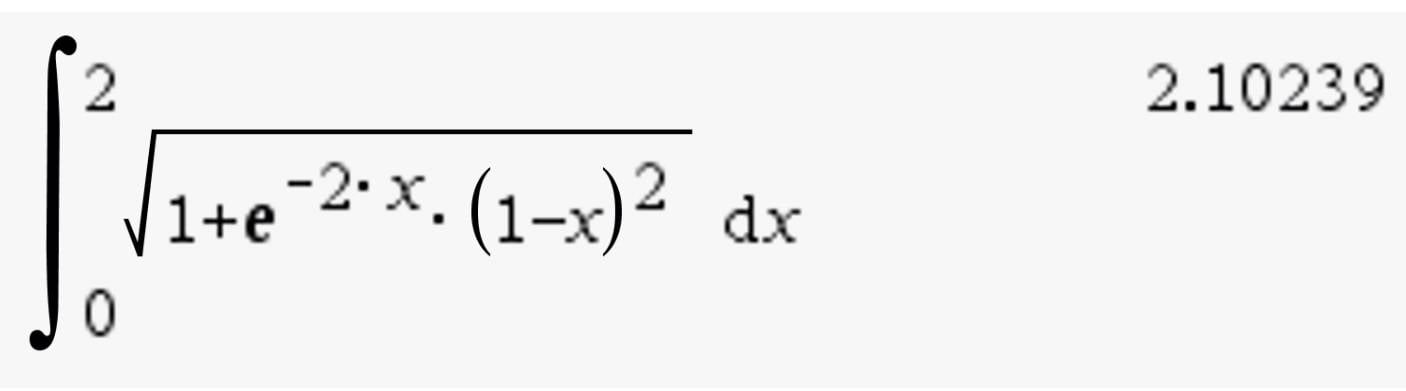# - Length of curve to 4 decimal pl

• MHB
• karush

#### karush

Gold Member
MHB
8.1.1
find the length of the curve to four decimal places
$y=xe^{-x},\quad 0 \le x \le 2$
eq from book
$L=\int_c^d\sqrt{1+[g'(y)]^2}\, dy$

ok I haven't done this in about 2 years and only did a few then so trying to review
rare stuff kinda

desmos graph

your cited book equation is for x as a function of y

the following is for the arclength of y as a function of x

$\displaystyle L = \int_0^2 \sqrt{1 + [e^{-x}(1-x)]^2} \, dx$what generated the image?

what generated the image?

TI Nspire program on my laptop

loaned out my TI Inspire
never got it back...If we are given that x= f(t), y= g(t), and z= h(t), for $t_1> t> t_0$ then the arclength is $\int_{t_0}^{t_1} \sqrt{f^{2}(t)+ g^{2}(t)+ h^2(t)}dt$.